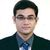# QlikView App Dev

Discussion Board for collaboration related to QlikView App Development.

Announcements
On May 18th at 10AM EDT we will answer your QlikView questions live. REGISTER
cancel
Showing results for
Did you mean:Creator III

## Define a range on Axes

Hi Experts,

I have a requirement to build a bar chart or any.

Requirement: Define a range on x-axis i.e. sum(value) for 0-199, 200-399, 400-599, 600-799, 800+

And that should be in an expression rather than in a script.

Note: I have fixed ranges.

Please find the attached qvd and the data is sample.

1 Solution

Accepted SolutionsMVP

Hi,

You should use expression like below.

=Aggr(if(sum(Value) >= 0 and sum(Value) < 200, '0-200',

if(sum(Value) >= 200 and sum(Value) < 400, '200-400',

if(sum(Value) >= 400 and sum(Value) < 600, '400-600',

if(sum(Value) >= 600 and sum(Value) < 800, '600-800', '800+')

)

)

),Field,SubField)

Regards,

Kaushik Solanki

Regards,
Kaushik
2 RepliesMVP

Hi,

You should use expression like below.

=Aggr(if(sum(Value) >= 0 and sum(Value) < 200, '0-200',

if(sum(Value) >= 200 and sum(Value) < 400, '200-400',

if(sum(Value) >= 400 and sum(Value) < 600, '400-600',

if(sum(Value) >= 600 and sum(Value) < 800, '600-800', '800+')

)

)

),Field,SubField)

Regards,

Kaushik Solanki

Regards,
Kaushik